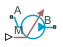# Flow Rate Source (2P)

Generate constant or time-varying mass flow rate or volumetric flow rate in two-phase fluid network

Since R2023b

•Libraries:
Simscape / Foundation Library / Two-Phase Fluid / Sources

## Description

The Flow Rate Source (2P) block represents an ideal mechanical energy source in a two-phase fluid network. The source can maintain the specified mass flow rate or volumetric flow rate regardless of the pressure differential. There is no flow resistance and no heat exchange with the environment. You specify the flow rate type by using the Flow rate type parameter.

The block icon changes depending on the values of the Source type and Flow rate type parameters.

Ports A and B represent the source inlet and outlet. The input physical signal at port M or V, depending on the flow rate type, specifies the flow rate. Alternatively, you can specify a fixed flow rate as a block parameter. A positive flow rate causes gas to flow from port A to port B.

### Mass Balance

The volume of fluid in the source is considered negligible and is ignored in a model. There is no fluid accumulation between the ports and the sum of all mass flow rates into the source must therefore equal zero:

`${\stackrel{˙}{m}}_{\text{A}}+\text{​}{\stackrel{˙}{m}}_{\text{B}}=0,$`

where $\stackrel{˙}{m}$ denotes the mass flow rate into the source through a port.

When you set the Flow rate type parameter to ```Volumetric flow rate```, the mass flow rate at port A is calculated from the volumetric flow rate:

where $\stackrel{˙}{V}$ is volumetric flow rate and v is specific volume.

### Energy Balance

By default, the source maintains the specified flow rate by performing isentropic work on the incoming fluid, though the block provides the option to ignore this term. The rate at which the source does work, if considered in the model, must equal the sum of the energy flow rates through the ports:

`${\varphi }_{\text{A}}+\text{​}{\varphi }_{\text{B}}+\text{​}{\varphi }_{\text{Work}}=0,$`

where ϕ denotes the energy flow rate into the source through a port or by means of work. The energy flow rate due to work is equal to the power generated by the source. Its value is calculated from the specific total enthalpies at the ports:

`${\varphi }_{\text{Work}}={\stackrel{˙}{m}}_{\text{A}}\left({h}_{\text{A}}-{h}_{\text{B}}\right).$`

The specific total enthalpy h is defined as:

`${h}_{*}={u}_{*}+{p}_{*}{v}_{*}+\frac{1}{2}{\left(\frac{{\stackrel{˙}{m}}_{*}{v}_{*}}{S}\right)}^{2},$`

where the asterisk denotes a port (A or B) and:

• u is specific internal energy.

• p is pressure.

• S is flow area.

The specific internal energy in the equation is obtained from the tabulated data of the Two-Phase Fluid Properties (2P) block. Its value is uniquely determined from the constraint that the work done by the source is isentropic. The specific entropy, a function of specific internal energy, must then have the same value at ports A and B:

`${s}_{\text{A}}\left({p}_{\text{A}},{u}_{\text{A}}\right)={s}_{\text{B}}\left({p}_{\text{B}},{u}_{\text{B}}\right),$`

where s is specific entropy. If the Power added parameter is set to `None`, the specific total enthalpies at the ports have the same value (${h}_{\text{A}}={h}_{\text{B}}$) and the work done by the source reduces to zero (${\varphi }_{\text{Work}}=0$).

### Assumptions and Limitations

• There are no irreversible losses.

• There is no heat exchange with the environment.

## Ports

### Input

expand all

Input physical signal that specifies the mass flow rate through the source.

#### Dependencies

To enable this port, set the Source type parameter to `Controlled` and Flow rate type to `Mass flow rate`.

Input physical signal that specifies the volumetric flow rate through the source.

#### Dependencies

To enable this port, set the Source type parameter to `Controlled` and Flow rate type to `Volumetric flow rate`.

### Conserving

expand all

Two-phase fluid conserving port. A positive flow rate causes fluid to flow from port A to port B.

Two-phase fluid conserving port. A positive flow rate causes fluid to flow from port A to port B.

## Parameters

expand all

Select whether the flow rate generated by the source can change during simulation:

• `Controlled` — The flow rate is variable, controlled by an input physical signal. Selecting this option exposes the input port M or V, depending on the value of the Flow rate type parameter.

• `Constant` — The flow rate is constant during simulation, specified by a block parameter. Selecting this option enables the Mass flow rate or Volumetric flow rate parameter, depending on the value of the Flow rate type parameter.

Select the type of flow rate, `Mass flow rate` or `Volumetric flow rate`.

Desired mass flow rate of the fluid through the source.

#### Dependencies

To enable this parameter, set Source type to `Constant` and Flow rate type to `Mass flow rate`.

Desired volumetric flow rate of the fluid through the source.

#### Dependencies

To enable this parameter, set Source type to `Constant` and Flow rate type to `Volumetric flow rate`.

Select whether the source performs work on the fluid flow:

• `Isentropic` — The source performs isentropic work on the fluid to maintain the specified mass flow rate. Use this option to represent an idealized pump or compressor and properly account for the energy input and output, especially in closed-loop systems.

• `None` — The source performs no work on the flow, neither adding nor removing power, regardless of the mass flow rate produced by the source. Use this option to set up the desired flow condition upstream of the system, without affecting the temperature of the flow.

Area normal to flow path at port A.

Area normal to flow path at port B.

## Version History

Introduced in R2023b

expand all